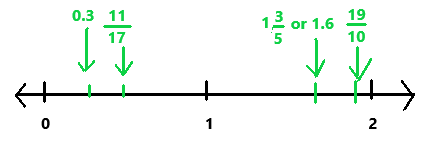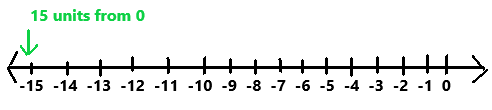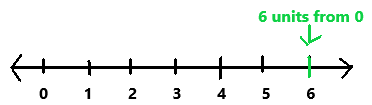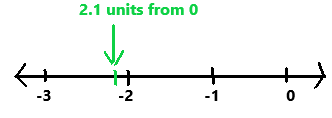# Math in Focus Grade 7 Chapter 1 Answer Key The Real Number System

Go through the Math in Focus Grade 7 Workbook Answer Key Chapter 1 The Real Number System to finish your assignments.

## Math in Focus Grade 7 Course 2 A Chapter 1 Answer Key The Real Number System

### Math in Focus Grade 7 Chapter 1 Quick Check Answer Key

Order the numbers from least to greatest. Use the < symbol. Graph each number on a horizontal number line.

Question 1.
$$\frac{11}{17}$$, 1$$\frac{3}{5}$$, 0.3, 1.6, $$\frac{19}{10}$$
$$\frac{11}{17}$$, 1$$\frac{3}{5}$$, 0.3, 1.6, $$\frac{19}{10}$$
The given numbers from least to greatest are 0.3 < $$\frac{11}{17}$$ < 1$$\frac{3}{5}$$  or 1.6 < 19/10.In the above image we can observe the given numbers on a horizontal number line.

Compare. Copy and complete each ? with <, > or =

Question 2.
3.87 ? 3.68
3.87 > 3.68
Explanation:
Compare the above given two decimal numbers. The two decimal numbers are 3.87 and 3.68. The decimal number 3.87 is greater than 3.68.

Question 3.
0.982 ? 0.982
0.982 = 0.982
Explanation:
Compare the above given two decimal numbers. The two decimal numbers are 0.982 and 0.982. The decimal number 0.982 is equal to 0.982.

Question 4.
5.23 ? 5.235
5.23 < 5.235
Explanation:
Compare the above given two decimal numbers. The two decimal numbers are 5.23 and 5.235. The decimal number 5.23 is less than 5.235.

Round each number.

Question 5.
1,456 to the nearest hundred.
Explanation:
1,456 is rounded to the nearest hundred as 1,500.
Explanation:
The number 1,456 is between 1,400 and 1,500. 1,450 is the midpoint between 1,400 and 1,500. The number 1,456 is greater than the midpoint. So, 1,456 is rounded to the nearest hundred as 1,500

Question 6.
849.58 to the nearest whole number.
849.58 is rounded to the nearest whole number as 850.
Explanation:
Here, the tenths value is 5 which is greater than or equal to 5, increase the whole number ones place by 1, and remove all the digits after the decimal point.
The number 849.58 becomes 850 after rounding to the nearest whole number.

Question 7.
4,923 to the nearest ten.
4923 is rounded to the nearest ten is 4920
When we are rounding to the nearest ten, we have to follow the below rules.
We have to round the number up. If the last digit in the number is 5, 6, 7, 8, or 9.
We have to round the number down. If the last digit in the number is 1, 2, 3, or 4.

Question 8.
23.84 to 1 decimal place.
23.84 is rounded to 1 decimal place is 23.8.
Explanation:
If the last digit in 23.84 is less than 5, then remove the last digit.
If the last digit in 23.84 is 5 or more and the second to the last digit in 23.84 is less than 9, then remove the last digit and add 1 to the second to the last digit.
If the last digit in 23.84 is 5 or more and the second to the last digit in 23.84 is 9, then remove the last digit, make the second to last digit 0, and add 1 to the number to the left of the decimal place.
The last digit in 23.84 is less than 5, So we have to remove the last digit. 23.84 is rounded to 23.8.

Question 9.
306.128 to the nearest hundredth.
306.128 is rounded to the nearest hundredth is 306.13.
Explanation:
If the digit after hundredth is greater than or equal to 5, then add 1 to hundredth. Else remove the digit.
The third digit of 306.128 right of decimal point is 8. Add 1 to the before decimal number then the number is 306.13.

Round 9,909.937 as indicated.

Question 10.
To 2 decimal places
9,909.937 is rounded to 2 decimal place is 9,909.9.
Explanation:
If the last digit after decimal point is greater than or equal to 5, then add 1 to before decimal number. Else remove the digit.
9,909.937 the third digit of right of decimal point is 7. Add 1 to the before decimal number then the number is 9,909.94
The second digit after decimal point is 4 which is less than 5.
So remove the number 4, the result is 9,909.9.

Question 11.
To the nearest whole number
9,909.937 is rounded to nearest whole number is 9,910.
Explanation:
Here, the hundredths value is 9 which is greater than 5. Increase the whole number ones place by 1, and remove all the digits after the decimal point.
9,909.937 after rounding to the nearest whole number becomes 9,910.

Question 12.
To the nearest whole number
9,909.937 is rounded to nearest whole number is 9,910.
Explanation:
Here, the hundredths value is 9 which is greater than 5. Increase the whole number ones place by 1, and remove all the digits after the decimal point.
9,909.937 after rounding to the nearest whole number becomes 9,910.

Question 13.
To the nearest ten
9,909.937 is rounded to nearest ten is 9,909.9.
Explanation:
If the digit after tenth is greater than or equal to 5, add 1 to tenth. Else remove the digit.
The third digit after decimal point is 7 which is greater than 5. Add 1 to the 3 which is second digit of the given number is 9,909.94.
The second digit after decimal point is 4 which is less than 5. So, remove the digits after 9.
The Result is equal to 9,909.9

Find the square of each number.

Question 14.
3
32
3 x 3 = 9
Explanation:
The given number is 3. Here we have to find the square of the given number. The square of number 3 is 9.

Question 15.
12
122
12 x 12 = 144
Explanation:
The given number is 12. Here we have to find the square of the given number. The square of number 12 is 144.

Find the cube of each number.

Question 16.
5
53
5 x 5 x 5 = 125
Explanation:
The given number is 5. Here we have to find the cube of the given number. The cube of number 5 is 125.

Question 17.
6
63
6 x 6 x 6 = 216
Explanation:
The given number is 6. Here we have to find the cube of the given number. The cube of number 6 is 216.

Find the square root and cube root of each number.

Question 18.
64
$$\sqrt{64}$$ = 8
3 √64 = 4
Explanation:
The square root of 64 is equal to 8.
The cube root of 64 is equal to 4.

Question 19.
729
$$\sqrt{729}$$ = 27
3 √729 = 9
Explanation:
The square root of 729 is equal to 27.
The cube root of 729 is equal to 9.

Order the numbers from greatest to least. Use the > symbol.

Question 20.
$$\sqrt{81}$$, 82, 33
$$\sqrt{81}$$ = 9
82 = 64
33  = 27
The numbers from greatest to least are 82, 33 ,$$\sqrt{81}$$.

Use the following set of numbers for questions 21 to 25.

34, -23, -54, 54, -60

Question 21.
Find the absolute value of each number.
The absolute values of the given numbers are as below.
The absolute value of |34| is 34.
The absolute value of |-23| is 23.
The absolute value of |-54| is 54.
The absolute value of |54| is 54.
The absolute value of |-60| is 60.

Question 22.
Which number is closest to 0?
The given set of numbers are 34, -23, -54, 54, -60
The number -23 is closest to 0.

Question 23.
Which number is farthest from 0?
The given set of numbers are 34, -23, -54, 54, -60.
The number -60 is farthest from 0.

Question 24.
Name two numbers with the same absolute value.
The given set of numbers are 34, -23, -54, 54, -60.
The absolute value of |-54| is 54.
The absolute value of |54| is 54.
The two numbers with the same absolute values are  -54 and 54.

Question 25.
Which number has the greatest absolute value?
The given set of numbers are 34, -23, -54, 54, -60.
The absolute value of |-60| is 60.
The number -60 has the greatest absolute value  as we can observe in the answer 21.

Use the number line to find the absolute value of each of the following numbers.

Question 26.
|-15|The given number is |-15|.
The absolute value of the number |-15| is 15.
So the number |-15| is 15 units from 0.

Question 27.
|6|The given number is |6|.
The absolute value of the number |6| is 6.
So the number |6| is 6 units from 0.

Question 28.
|-2.1|The given number is |-2.1|.
The absolute value of the number |-2.1| is 2.1.
So the number |-2.1| is 2.1 units from 0.

Copy and complete each ? with >, =, or <.

Question 29.
|-7| ? |-72|
|-7| < |-72|
Explanation:
The absolute value of |-7|  is 7.
The absolute value of |-72|  is 72.
So, |-7| is less than  |-72|.

Question 30.
|5| ? |-5|
|5| = |-5|
Explanation:
The absolute value of |5| is 5.
The absolute value of |-5| is 5.
So, |5| is equal to |-5|.

Question 31.
|-26| ? |5|